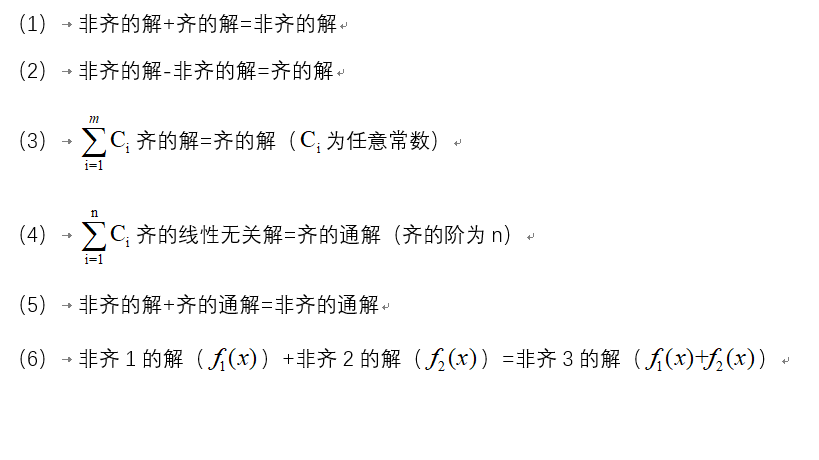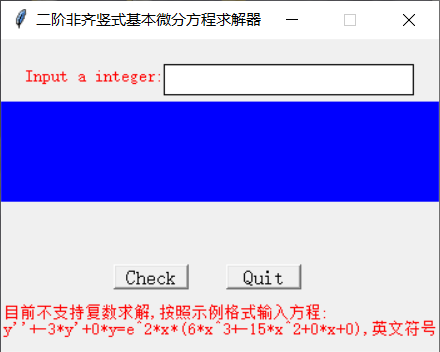• 用Matlab解二阶非齐次微分方程大纲函数代码 大纲 用Matlab解二阶非齐次微分方程，网上很多麻烦又累赘又无用的东西，一句话解决的事。 函数 dsolve(‘a’，‘b’，‘c’)：解微分方程函数 a：（二阶）微分方程 b： ...
用Matlab解二阶非齐次微分方程大纲函数代码
大纲
用Matlab解二阶非齐次微分方程，网上很多麻烦又累赘又无用的东西，一句话解决的事。
函数
dsolve(‘a’，‘b’，‘c’)：解微分方程函数
a：（二阶）微分方程
b：  初值，可不填
c：  自变量
代码
a=dsolve('D2y+2*Dy+5*y=sin(2*t)','t')
simplify(a)



展开全文matlab
• 不要嘲笑我，我百度经验搬过来的二阶常系数线性非齐次方程的形式如下2基本求解思路如下，我们先要有一个总的思路用于解题3难点：对于特解的求法END一.特解求法一：待定系数法1优点：简单易懂，不易错缺点：计算量...
不要嘲笑我，我百度经验搬过来的二阶常系数线性非齐次方程的形式如下2基本求解思路如下，我们先要有一个总的思路用于解题3难点：对于特解的求法END一.特解求法一：待定系数法1优点：简单易懂，不易错缺点：计算量相比其他较大2总的思路如下：3二阶常系数非齐次方程的非齐次项f(x)与特解的关系如下，该表需要记住才能用于解题。4这里小编把例题的详细过程已经写出来了，一般辅导书不会有详细的解答，如果还没有明白，请联系小编。END一.特解求法二：微分算子法1优点：简单快捷，适合处理2次以上微分方程2要学习微分算子，我们先引入以下几个符号3那么，该如何表示特解呢？4不知道同学们能否理解？其实不理解也没关系，和小编一起做下面这道题基本就没问题了。这道题{}符号里的在具体解题时不用写出，因为那是小编为了你们更好理解才写出来的，公式的变化和推导要多做才会。5所以上面一题原方程的通解为展开全文• 二阶线性非齐次微分方程非齐 重要的性质、定理（共6条）： 证明1：若y1、y2、y3是非齐的解，a、b、c为常数且a+b+c=0，y=a y1+by2+cy3则y是齐的解 由(7) ，则需要证明a y1+by2+cy3是 齐的解。 因为y1、...
以下简称

二阶线性齐次微分方程 为 齐
二阶线性非齐次微分方程 为 非齐

重要的性质、定理（共6条）：证明1：若y1、y2、y3是非齐的解，a、b、c为常数且a+b+c=0，y=a y1+by2+cy3则y是齐的解

由(7)  ，则需要证明a y1+by2+cy3是 齐的解。

因为y1、y2、y3是非齐的解，故y1－y2、y2-y3是齐的解，则
C1（y1－y2）+C2（y2-y3）也是齐的解
故C1（y1－y2）+C2（y2-y3）就是齐的解
显然y1的系数=C1=a
y2的系数=-C1+C2=b
y3的系数=-C2=c
a+b+c=0

证明2： 若y1、y2、y3是非齐的解，a、b、c为常数且a+b+c=１，y=a y1+by2+cy3 则y是非齐的解

由（1），则需要证明a y1+by2+cy3是非齐的解＋齐的解

因为y1、y2、y3是非齐的解，故y1－y2、y2-y3是齐的解，则
C1（y1－y2）+C2（y2-y3）也是齐的解，而y3是非齐的解，
故C1（y1－y2）+C2（y2-y3）+y3就是非齐的解
显然y1的系数=C1=a
y2的系数=-C1+C2=b
y3的系数=-C2+1=c
a+b+c=1

证明3：若y1、y2、y3是非齐的线性无关解，a、b、c为任意常数且a+b+c=0，

y=a y1+by2+cy3，则y是齐的通解
由（7）
y1、y2、y3是非齐的线性无关解，现假设y1-y2、y2-y3也是线性无关的，
则齐的通解y=C1(y1-y2)+C2(y2-y3)
显然y1的系数=C1=a
y2的系数=-C1+C2=b
y3的系数=-C2=c
a+b+c=0

补充证明：现证明y1-y2、y2-y3是线性无关的。

假设线性有关，则存在不全为0的k1和k2，
使得k1（y1-y2）+k2（y2-y3）恒等于0
即：
(k1)y1+(k2-k1)y2-(k2)y3恒等于0
由于y1、y2、y3是线性无关的，故k1=0、k2=0，与假设相违背。因此，y1-y2、y2-y3是线性无关的。

证明4：若y1、y2、y3是非齐的线性无关解，a、b、c为任意常数且a+b+c=1，y=a y1+by2+cy3，则y是非齐的通解

由（5）
y1、y2、y3是非齐的线性无关解，现假设y1-y2、y2-y3也是线性无关的，
则齐的通解y=C1(y1-y2)+C2(y2-y3) ，
非齐的解为y3（你换成y1或者y2都是一样的，因为最后大家系数都是要加在一起的）
显然y1的系数=C1=a
y2的系数=-C1+C2=b
y3的系数=-C2+1=c
a+b+c=1
展开全文线性
• #coding = 'utf-8' import tkinter as tk class Root(object): def __init__(self,root): self.root = root self.root.title("二阶非齐竖式基本微分方程求解器") self.root.geometry("350x250") self.root....
TIPS
算法使用python编写，基本上除了GUI库，不使用任何第三方数学库．
关于竖式解法请参考如下视频：
链接：https://pan.baidu.com/s/1VynxkqseUBt9Abi9k4K-CA
提取码：6666
代码
代码部分不多比比，很多变量都是直接使用中文拼音写的，不存在看不懂的问题．如果看不懂，请仔细查看上面的解法链接，或者复习python．
#coding = 'utf-8'
import tkinter as tk
class Root(object):
def __init__(self,root):
self.root = root
self.root.title("二阶非齐竖式基本微分方程求解器")
self.root.geometry("350x250")
self.root.resizable(width=False,height=False)
self.tips = tk.StringVar()
def Label(self):
Label_IN = tk.Label(self.root,text="Input a integer:",fg='red',font=("宋体",10))
Label_IN.place(x=20,y=20,width=110,heigh=20)
tips_code = '''目前不支持复数求解,按照示例格式输入方程:\ny''+-3*y'+0*y=e^2*x*(6*x^3+-15*x^2+0*x+0),英文符号'''
Label_TIPS = tk.Label(self.root,font=("宋体",10),text=tips_code,justify='left',fg='red')
Label_TIPS.place(x=0,y=205,width=350,height=40)
self.IN_Get = tk.Entry(self.root,font=('宋体', 12),fg='gray',relief='solid',textvariable=self.tips)
self.IN_Get.place(x=130,y=20,height=25,width=200)
self.Show_AN = tk.Label(self.root,fg='red',font=("宋体",10),bg='blue')
self.Show_AN.place(x=0,y=50,height=80,width=350)
self.Check_B = tk.Button(self.root,text='Check',font=("宋体",12))
self.Check_B.place(x=90,y=180,height=20,width=60)
self.Quit_B = tk.Button(self.root,text='Quit',font=("宋体",12))
self.Quit_B.place(x=180,y=180,height=20,width=60)
class Tools(object):
def QiuDao_F(self,nn):
if "*" in nn and "^" in nn:
l1 = nn.split('*')
l2 = nn.split('^')
get_s = str(float(l1)*float(l2))+'*x'+'^'+str(float(l2)-1)
return get_s
else:
if '*' in nn:
get_s = nn.split('*')
return get_s

elif '^' in nn:
l2 = nn.split('^')
get_s = l2+'*x^'+str(float(l2)-1)
return get_s
elif nn == 'x':
return '1'
else:
return '0'

def JianDao_F(self,a,b):
if '*' in a and '*' in b:
l1 = a.split('*')

l2 = b.split('*')
l3 = str(float(l1)-float(l2))+'*'+a.split('*')
return l3
else:
return str(float(a)-float(b))
def ChuDao_F(self,x,lmd):
if 'x'==x:
return str(1/lmd)
if '*' in x:
l1 = x.split('*')
l2 = '{:,.2f}'.format(float(l1)/lmd)
get_s = l2+'*'+l1
return get_s
else:
return '{:,.2f}'.format(float(x)/lmd)

def GET_GO(self,Need_Do,lmd):
global star, g, end
g = ''
nn = Need_Do.split('+')
star = 0
end = len(nn)-1
ll1=self.ChuDao_F(nn,lmd)
g+=ll1
global star,g,end
if star==end:
return g
ll2=self.QiuDao_F(ll1)
ll3=self.JianDao_F(nn[star+1],ll2)
ll4=self.ChuDao_F(ll3,lmd)
g+='+'+ll4
star+=1
return g

def GetABC(x):
if '*' not in x:
return 1.0
else:
return float(x.split('*'))

def ANSW_GET(y):
Tool = Tools()
QiChi = y.split('=')
FeiQiChi = y.split('=')
R_E  = FeiQiChi.split('(')
if R_E:
Lmd = R_E.split('^')
Lmd = float(Lmd.split('*'))
else:
Lmd = 0

Need_Do = FeiQiChi.split('(').rstrip(')')

a,b,c = map(GetABC,QiChi.split('+'))
def TUX(a,b,c):
N = (b**2)-4*(a*c)
if N>=0:
return (N**0.5)
else:
return 0

Tux = TUX(a,b,c)
if Tux:
x1 = (-b+Tux)/(2*a)
x2 = (-b-Tux)/(2*a)
if x1 == x2:
Omg = Lmd - x1
g = Tool.GET_GO(Need_Do,Omg)
s = 'y*='+R_E+'('+g+')'+'\nx = {}'.format(x1)
return s
else:
Omg1 = Lmd - x1
Omg2 = Lmd - x2
if Omg1 <= Omg2:
Omgp = Omg1
Omg1 = Omg2
Omg2 = Omgp
g0= Tool.GET_GO(Need_Do, Omg1)
g = Tool.GET_GO(g0,Omg2)
s ='y*='+ R_E + '(' + g + ')'+'\nx1 = {},x2 = {}'.format(x1,x2)
return s

else:
return 0
def Check_Go(xincan):
print(Win.IN_Get.get())
y = Win.IN_Get.get()
s = ANSW_GET(y)
if s:
Win.Show_AN.configure(text=s,font=("宋体",10))
else:
Win.Show_AN.configure(text='抱歉目前不支持此类方程求解',font=("宋体",15))
def Quit_Go(xincan):
Win.Show_AN.configure(text='',font=("宋体",10))
Win.tips.set('')
Win.IN_Get.config(fg='black', textvariable=Win.tips)

if __name__=='__main__':
root=tk.Tk()
Win = Root(root)
Win.Label()
Win.Check_B.bind("<Button-1>",lambda a :Check_Go('0'))
Win.Quit_B.bind("<Button-1>", lambda a: Quit_Go('0'))
root.mainloop()


效果展开全文python tkinter 算法
• 二阶线性齐次微分方程边值问题相似构造解的应用及Matlab图版分析.pdf
• 二阶线性齐次微分方程边值问题相似构造解式的基础上，首先利用相似构造法求解Bessel方程和变型的Bessel方程边值问题的解，然后建立了均质油藏的渗流规律的数学模型，再将均质油藏的渗流数学模型转换成变型的Bessel...
• 一般二阶线性非齐次微分方程的解与对应齐次方程的解的关系@(微积分)设p(x),q(x),f(x),f(x)≠0p(x),q(x),f(x),f(x)\neq 0为连续函数，对于下面的二阶线性非齐次方程：y″+p(x)y′+q(x)y=f(x)y''+p(x)y'+q(x)y = f(x) ...
• 二阶常系数非齐次微分方程的复数解法二阶常系数非齐次微分方程的复数解法二阶常系数非齐次微分方程的复数解法二阶常系数非齐次微分方程的复数解法
• 提出一类二阶n次齐微分方程的可积充分条件，给出了它的通解表达式，并列举若干实用的推论。
• 通过对二阶齐次线性微分方程边值问题的解析表达式进行整理和简化，得到了解式的相似结构形式；说明了该类微分方程的解，具有类似于实数可表为连分式，图形具有相似性的所谓式相似性质；指出了解式的相似性质的研究，...
• 好难啊，第十期还没有更，十一期的也没时间了，明天满课，还有个视频要做，再占个坑，如果计划顺利，11期是二阶线性非齐次微分方程的解。嗯，加油。 ...
• 对一类形如x2y″+pxy′+qy=f(x)的二阶欧拉非齐次线性常微分方程,利用变量变换化为常系数线性常微分方程。然后用复数法讨论了具有形如f(x)=Axαcos(βln|x|)和f(x)=Axαsin(βlnx)非齐次项时求特解的方法,得到了用A F...
• 人生有无数的可能性，考研的结果一定不是终点!但做的每一个选择都要坚持到最后!这是对自己、对梦想最大的尊重!用探索方法代替消极迷茫，用寻求技巧抵消杂乱慌张!争分夺秒，竭尽所能!悉心浇灌，静候花开!...数学
• 1、二阶常系数齐线性微分方程 2、二阶常系数非齐次线性微分方程
• 提出并证明了二阶常系数线性非齐次微分方程y″+py′+qy=Pm(x)eλx的特解定理，给出了特解公式．
• 二阶方程再也不是一个梦想，在20世纪已经有人能解开齐次，而我们
• 讨论了一类二阶线性微分方程解的振动性质，建立了两个新的振动性定理，推广和改进了Grace和Lalli（J Math Anal Appl，1987，123：584-588）的结果。
• 给出了二阶常系数线性非齐次微分方程y+ay1+by=f（x）的多种解法，旨在介绍二阶方程的一些解题方法与解题思路。
• 例：求y′′+y=cos2x+2sinx的通解例：求 y&amp;#x27;&amp;#x27;+y= cos{2x}+2sinx 的通解例：求y′′+y=cos2x+2sinx的通解 ...∴将方程式y′′+y=cos2x+2sinx\therefore 将方程式 y&amp;#x27;&amp...
• 二阶齐次线性微分方程 解的叠加 这样的处理可以让解的形式更加简洁，也便于理解的叠加原理 线性相关与线性无关 注：以下用到线性代数的知识，但这个我是上个学期自学的，可能会有错误。 就记两条准则来判断线性...
• 利用格林函数的正性和Krasnoselskii不动点定理建立了二阶奇异线性微分方程周期边值问题解的存在性和多重性结果。当线性项f具有奇性且线性时，方程至少存在一个正解；当f具有奇性且超线性时，方程至少存在两个...
• 二阶齐线性微分方程就是指形如y′′+py′+q=0y&#x27;&#x27;+py&#x27;+q=0y′′+py′+q=0的微分方程，一种解法就是使用二阶微分方程的公式，这里介绍另一种方法。 对于二阶微分方程，如果找到两个线性...
• 这是二阶微分方程，可以求得通解。 这里的常数是有意义的，表示Z=0处入射电压和反射电压。 信号传输入点我们一般认为在z<0处， Assume that an incident wave of the form V0+e−γzV_0^+e^{-{\gamma}z}V0+​e...
• 二阶常系数非齐次线性微分方程的通解 二阶常系数非齐次线性微分方程的形式为： ay″+by′+cy=f(x) 微分方程的通解 = 对应的二阶常系数齐线性微分方程通解 + 自身的一个特解 简单记为：通解 = 齐通解 + ......